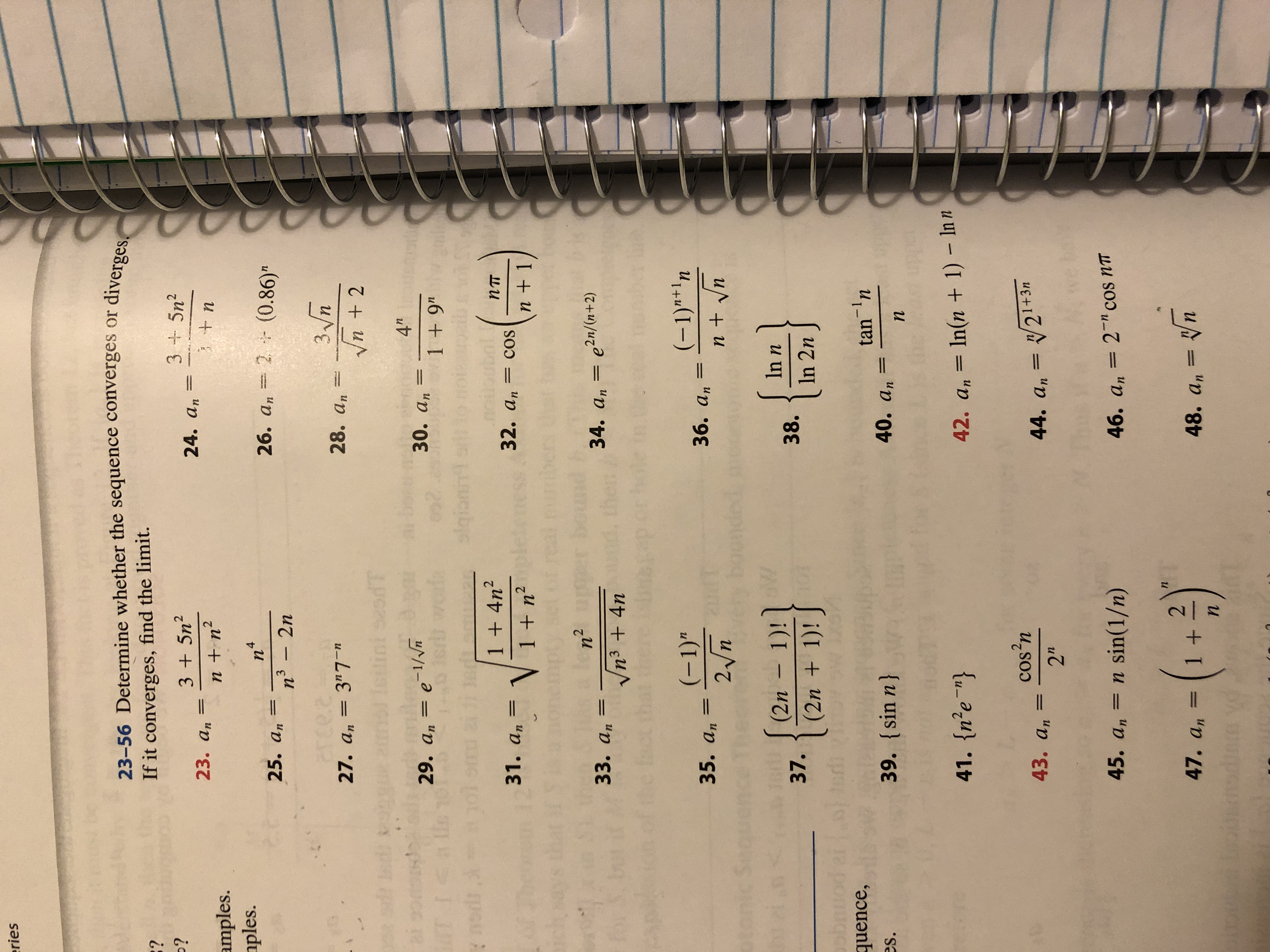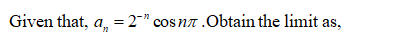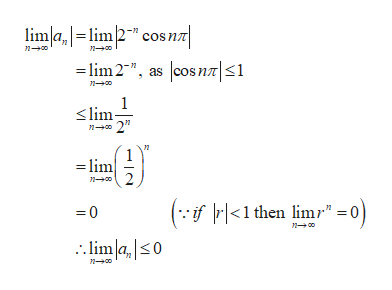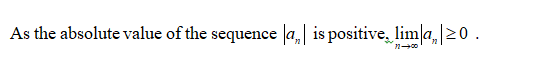# riesdiverges23-56 Determine whether the sequence converges orIf it converges, find the limit.:??3 +5n23 5n224. an23. аn+nnn2amples.ples.n426. an 2(0.86)"25. ann3 2n3nVn22ce.e27. an 3"728. antini sodT4"30. аn29. а, — е 1/1 + 9"4 IStnodrsai1 + 4n2пт32. an=cos31. а,Vmen + 11 + n2boundn2n3 4ne2n/(a+2)34. an e2n/(n+2)3. аn(-1)"1n(-1)"36. аn35. аnn + n2 nboondootoSeoSW(2n 1)10t(2n 1)!{In n38.37.In 2nquence,tan n40. аn39. sin n}es.пIn(n +1)- In n41. {n'e"}42. ancos'nV21+3m44. an43. аn2b2 " cos nT45. а,= n sin(1/n)46. an- ()n21 +47. an48. аnn

Question
2 views

Determine whether the sequence converges or diverges. If it converges, find the limit.

46. a sub n = (2^-n)cos n pihelp_outlineImage Transcriptioncloseries diverges 23-56 Determine whether the sequence converges or If it converges, find the limit. :? ? 3 +5n2 3 5n2 24. an 23. аn +n nn2 amples. ples. n4 26. an 2(0.86)" 25. an n3 2n 3n Vn2 2ce.e 27. an 3"7 28. an tini sodT 4" 30. аn 29. а, — е 1/ 1 + 9" 4 IS tnodr sai 1 + 4n2 пт 32. an=cos 31. а, V me n + 1 1 + n2 bound n2 n3 4n e2n/(a+2) 34. an e2n/(n+2) 3. аn (-1)"1n (-1)" 36. аn 35. аn n + n 2 n boondo oto Seo SW (2n 1) 10t (2n 1)! { In n 38. 37. In 2n quence, tan n 40. аn 39. sin n} es. п In(n +1)- In n 41. {n'e"} 42. an cos'n V21+3m 44. an 43. аn 2 b 2 " cos nT 45. а, = n sin(1/n) 46. an - () n 2 1 + 47. an 48. аn n fullscreen
check_circle

Step 1help_outlineImage Transcriptioncloselimlalim2" cos n lim2", as cosnT| 1 n0 n00 1 lim n 2" lim n 2 (-f<1 then limr" =0) 0 no limla,s0 n-00 fullscreen
Step 2...

### Want to see the full answer?

See Solution

#### Want to see this answer and more?

Solutions are written by subject experts who are available 24/7. Questions are typically answered within 1 hour.*

See Solution
*Response times may vary by subject and question.
Tagged in

### Other